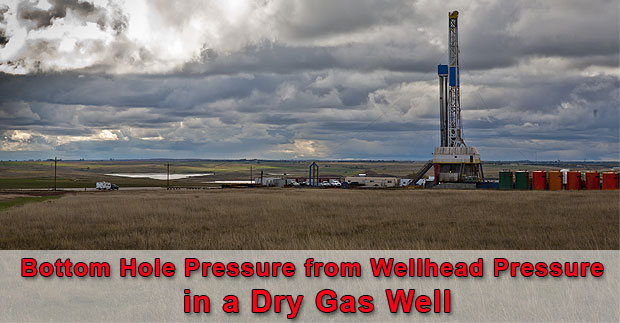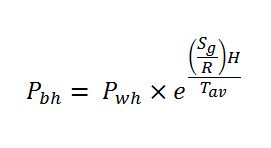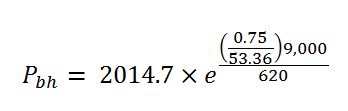# Determine Bottom Hole Pressure from Wellhead Pressure in a Dry Gas Well

Gas behaves differently from fluid therefore you cannot use a simple hydrostatic formula to determine reservoir pressure. Gas is compressible but fluid is incompressible.The formula to determine the bottom hole pressure of dry gas well is shown below;Where; Pbh = bottom hole pressure in psia (absolute pressure)

Pwh = wellhead pressure in psia (absolute pressure)

H = true vertical depth of the well

Sg = specific gravity of gas

R = 53.36 ft-lb/lb-R (gas constant for API standard condition air)

Tav = average temperature in Rankin (Rankin = Fahrenheit + 460)

Example: The dry gas well is shut in and the well head pressure is 2,000 psig (gauge pressure). The average wellbore temperature is 160 F. Gas specific gravity is 0.75. The well is 9,000’ TVD and the wellhead is on land. Determine the bottom hole pressure and compare the result if you use a normal relationship from hydrostatic pressure calculation.

Pwh = 2,000 + 14.7 = 2,014.7 psia

H = 9,000 TVD

Sg = 0.75

Tav = 160 + 460 = 620 °RPbh = 2,471 psia

Pbh = 2,471 – 14.7 = 2,456 psig

If you use hydrostatic pressure calculation, the bottom hole pressure is calculated by the following equation.

Pbh = Pwh + (0.052 x average gas density (ppg) x TVD of the well, ft)

Average air density at 160 F is 6.404 x 10-2  (lb/ft3) = 8.56 x 10-3 ppg

Average gas density at 160 F = gas specific gravity x Average air density at 160 F

Average gas density at 160 F = 0.75 x  8.56 x 10-3 ppg = 6.42 x 10-3 ppg

Pbh = 2000 + (0.052x6.42 x 10-3x9,000)= 2003 psia

As you can see from the calculation, the hydrostatic pressure cannot be used to determine the bottom hole pressure of the dry gas well. It will give you inaccurate result.

Share the joyWorking in the oil field and loving to share knowledge.

### 4 Responses to Determine Bottom Hole Pressure from Wellhead Pressure in a Dry Gas Well

1.ahmed ibraheem says:

there is a mistake in answer
Pbh = 2,471 – 14.7 = 2,456 psia you have to change the unit to psig

•DrillingFormulas.Com says:

Ahmed,

Thanks for informing us. This is our typo mistake and it is taken care of.

Regards,
Shyne

2.Bennezzar says:

3.Lashar Lavenue says: